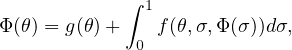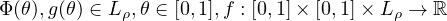Electron. J. Differential Equations, Vol. 2019 (2019), No. 36, pp. 1-9.

### Nonlinear Fredholm equations in modular function spaces Mostafa Bachar

Abstract:
We investigate the existence of solutions in modular function spaces of the Fredholm integral equationwhere. An application in the variable exponent Lebesgue spaces is derived under minimal assumptions on the problem data.

Submitted March 13, 2018. Published March 5, 2019.
Math Subject Classifications: 46A80, 47H10, 45G05.
Key Words: Electrorheological fluids; fixed point; Fredholm equations; modular function spaces; variable exponent spaces.

Show me the PDF file (322 KB), TEX file for this article.Mostafa Bachar College of Sciences Department of Mathematics King Saud University Riyadh, Saudi Arabia email: mbachar@ksu.edu.sa